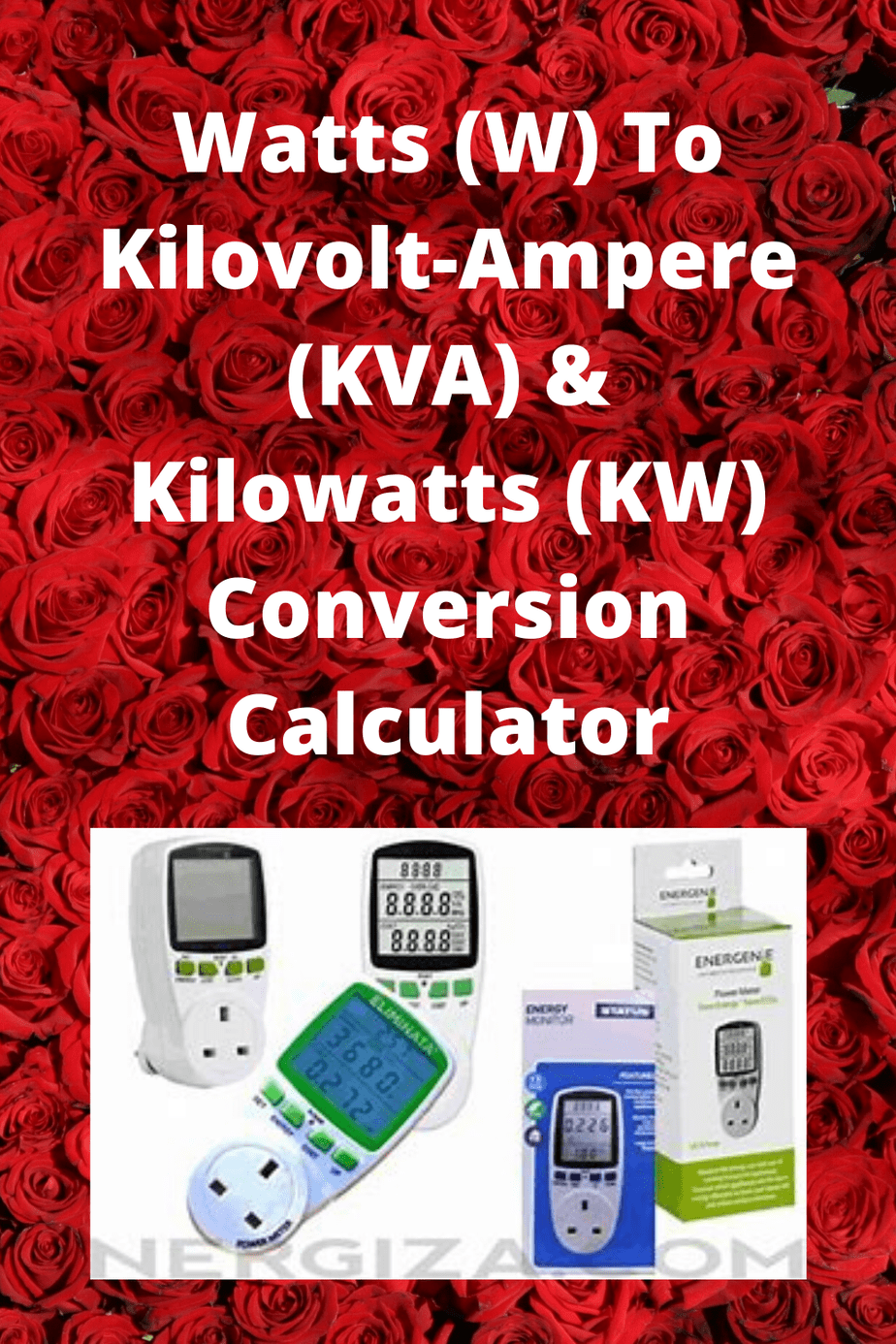# Watts (W) To Kilovolt-Ampere (KVA) & Kilowatts (KW) Conversion CalculatorCheck out Best generators for home use on AmazonCheck out best generator for home use on AmazonDisclaimer: Every time, it comes to electric and power calculations, it is a good idea to get advice from an expert for your specific situation. The calculations on this web page are for general estimations only.

Watts to kVA calculator

The watt (symbol “W”) is a unit term of power and often used in specification from various kinds of electrical appliances.

It is defined in the International System of Units (SI) as the amount of energy (symbol: J) transfer per second (symbol: s), no matter the energy is generated or dissipated during the process.

W = J/s

Further elaboration can also be utilized to demonstrate watts as follows. When an object possesses fixed or constant velocity at one meter per second against a constant opposing force of one newton (symbol: N), the rate of work exerted is defined as one watt.

W = J/s = Nm/s = kgm*m/s

Before moving on to Watts to kVA calculator, there are two terms should be introduced first. First is apparent power (symbol: S), which is the product of root-mean-square (RMS) voltage and RMS current.

The term becomes crucial during the design phase of electrical systems. Another term is power factor (symbol: PF), which is defined by the ratio of the real power absorbed or utilized by the load to the apparent power entering electrical systems.

The higher the ratio, the less the current drawn by the electrical systems, indicating lower energy loss and smaller wires requirement for the electrical systems.

The volt-ampere (VA) is a unit term for the apparent power (S) in an electrical system, just like watts for power.

It is defined as the power in watts, divided by 1000 times the power factor as follows.

Under the same amount of power transferred during the process, the higher the power factor, the lower the apparent power. kVA is 1000 times of VA.

S = W/PF (S unit in VA)

S = W/(1000xPF) (S unit in kVA)

Hence, in the theoretical electrical system, apparent power should be equal to actual power. In reality, numerous loads in the electrical system exhibit power dissipation.

Check out Best generators for home use on AmazonThus, it’s crucial to utilize Watts to kVA calculator to specifically derive the apparent power of a certain device to understand its capability and efficiency in electrical performance.

Related Articles

Free and fast generator run time calculator online